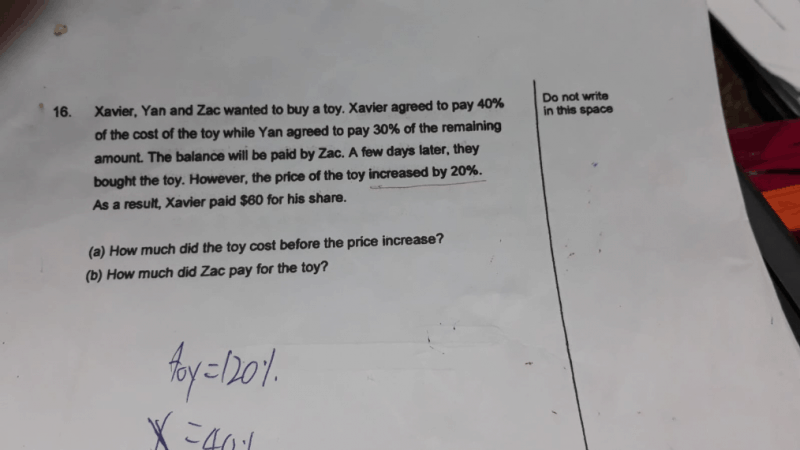# Questiona) Xavier: 40% of final cost

Yan: 30% of the remaining 60%
= 30/100 x 60%
= 18% of final cost

Zac: 100% – 40% – 18%
= 42% of final cost

40% of new price → \$60
10% of new price →  \$15
100% of new price → \$150

100% of new price = 120% of original price = \$150

120% of original price → \$150
10% of original price → \$12.50
100% of original price → \$125

b) 42% of final cost 42/100 x \$150
= \$63

Check

 Share (%) Share (\$) for original price Share (\$) for new price Xavier 40% 40% x \$125 = \$50 40% x \$150 = \$60 Yan 18% 18% x \$125 = \$22.50 18% x \$150 = \$27 Zac 42% 42% x \$125 = \$52.50 42% x \$150 = \$63 Total 100% \$125 \$150
0 Replies 0 Likes